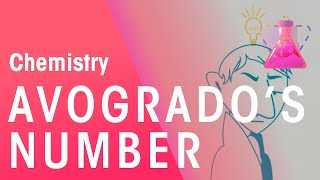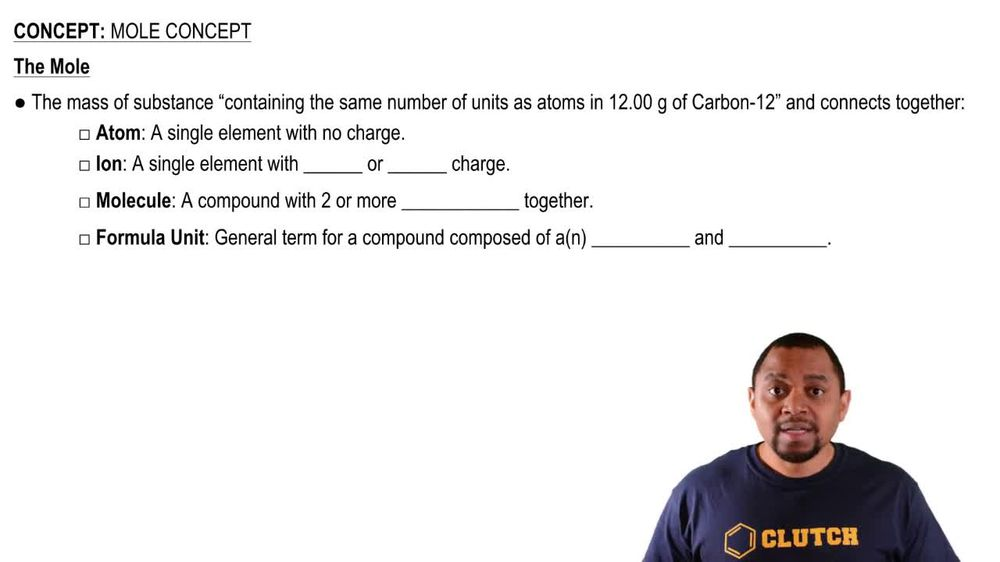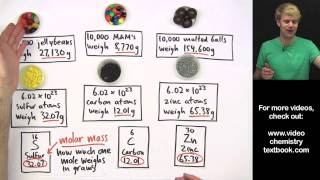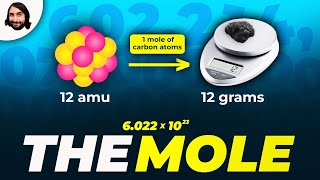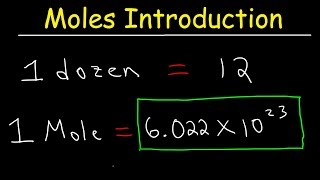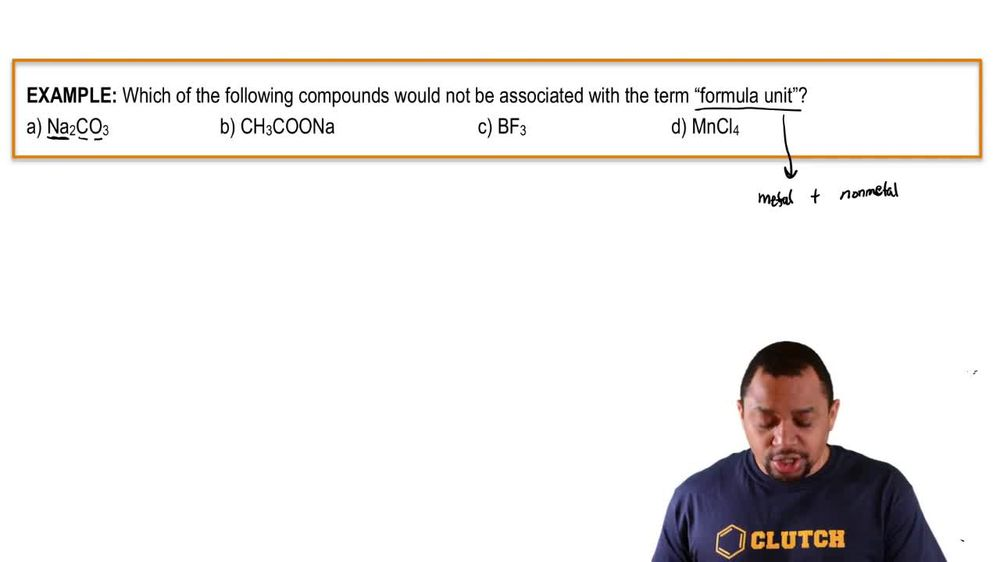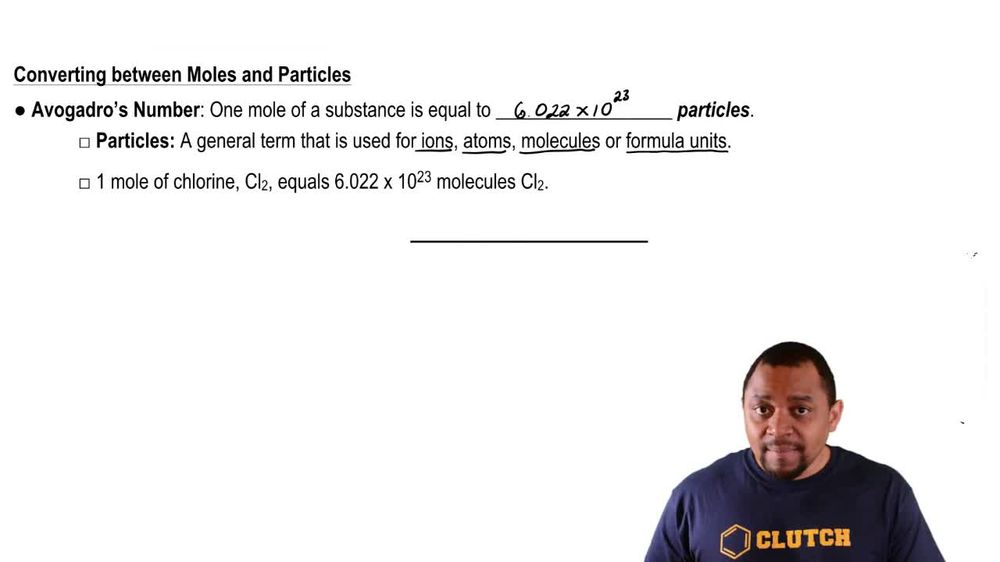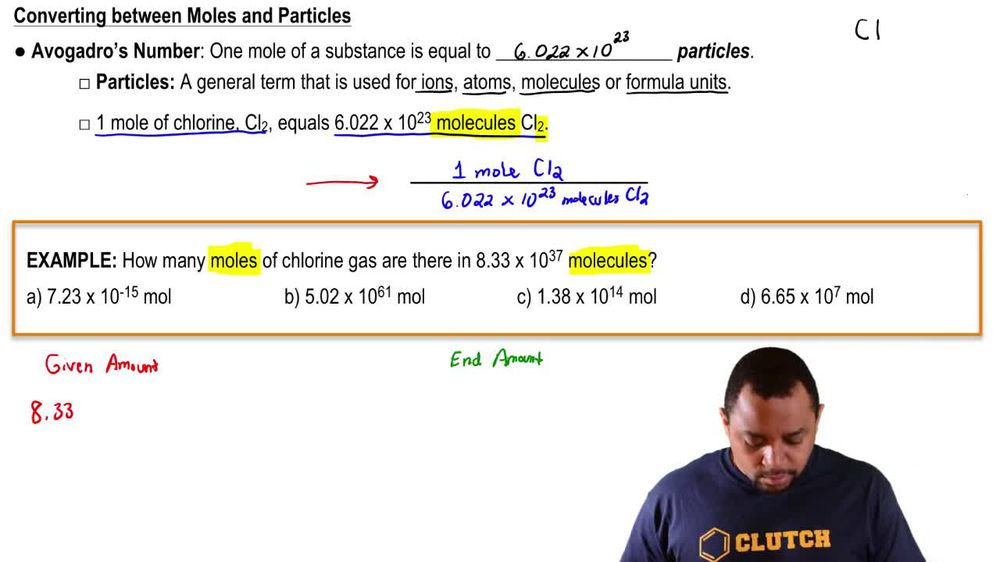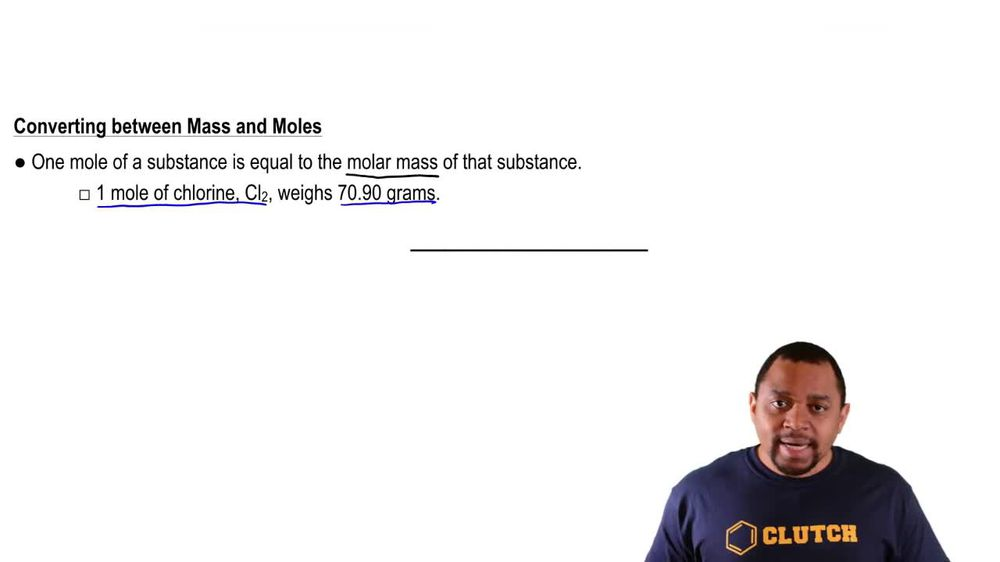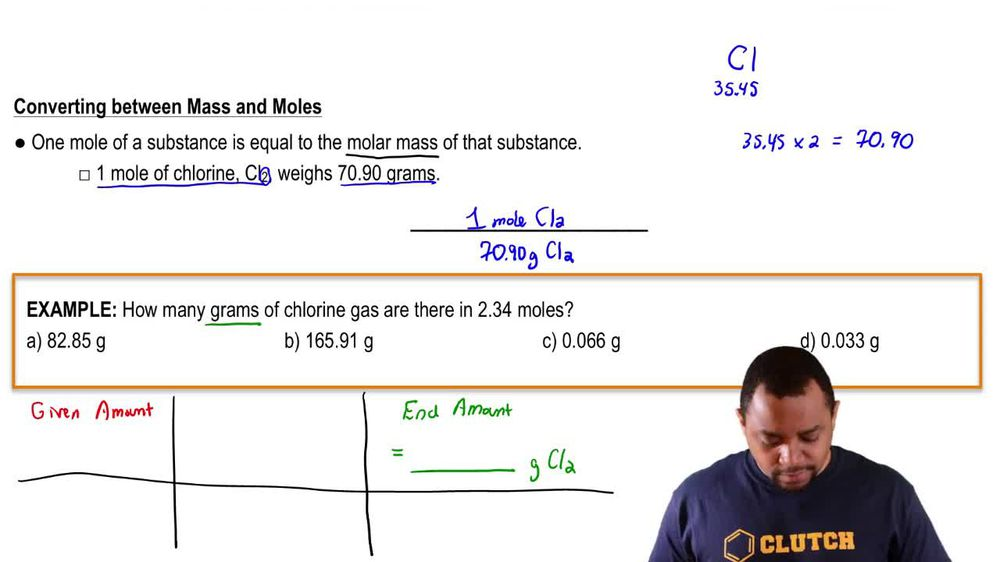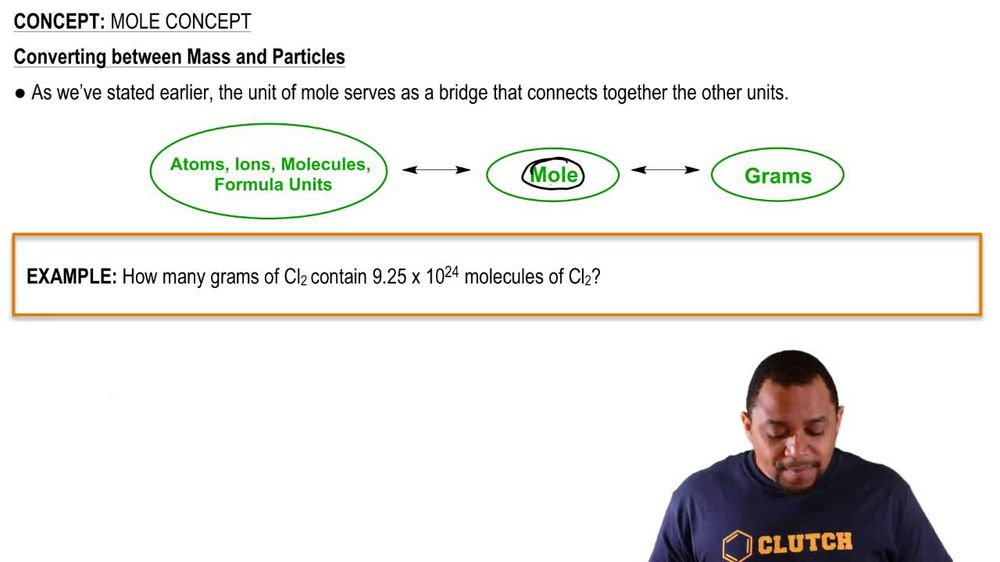Start typing, then use the up and down arrows to select an option from the list.
1. 2. Atoms & Elements2. Mole Concept
Problem

# How many grams are in a mole of each of the following substances? (d) H2O

Relevant Solution59s
Play a video:
Hi everyone here we have a question asking if a statement is true or false, one mole of water has a mass of 18.02 g. So we're going to add up the molar mass of H20. So we have two hydrogen With a mass of 1.01 grams per mole plus one oxygen, Which has a mass of 16 g per mole, And that equals 18 . g Permal. So this statement is true, and that is our final answer. Thank you for watching. Bye.